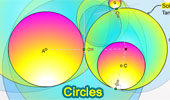# Online Math: Geometry: Circles, Chord, Diameter, Arc, Semicircle, Tangent, Theorems and Problems, High School, College

## Circles Index (Page 1)Geometric Art: Circle 2. iPad Apps.
Fractal Image with Circle.

Common Chord to two circles.
Theorems and Problems.

Geometric Art: Circle. iPad Apps: fractBG.
Fractal Image with Circle.

Chord. Index.
A chord of a circle is the line segment joining two points on the circle.

Perpendicular Chords. Index.
Theorems and Problems.

Semicircle. Index.
Theorems and Problems.

Circular Sector. Index.
Theorems and Problems.

Circular Segment. Index.
Theorems and Problems.

Circle Tangent Line. Index.
Theorems and Problems.

Circle Area. Index.
Theorems and Problems.

Concentric Circles. Index.
Theorems and Problems.

Intersecting Circles Index.
Theorems and Problems.

Arbelos, Theorems and Problems
Three tangent semicircles with collinear centers.
Index

Apollonius of Perga, Theorems and Problems
Index

Circumcircle Index.

GeoGebra, Dynamic Geometry: Nine Point Circle
HTML5 Animation for Tablets (iPad, Nexus..)

Circle Inscribed in a Square, Theorems and Problems. Index.

Butterfly theorem proof
HTML5 Animation for iPad and Nexus.

GeoGebra, Dynamic Geometry, Euclidean Egg with 8 Arcs in 5 Steps, Step-by-Step Construction
HTML5 Animation for Tablets (iPad, Nexus)

Go to Page: Previous | 1 | 10 | 20 | 30 | Next

Home | Sitemap | Search | Geometry | Circle | Email | by Antonio Gutierrez
Last updated: Oct 13, 2019